office (412) 9679367
email: ftsweb@gmail.com
toll-free 1 (800) 214-3480

4.4 Treasury Bills

 T

hree types of Treasury bills (T-bills) are issued with the following short maturities: 13 weeks (91 days), 26 weeks (182 days), and 52 weeks (365 days). The 13- and 26-week T-bills are auctioned by the Treasury every week on Mondays (with adjustments made for public holidays) and issued on the following Thursday. The 52-week T-bills are auctioned every fourth Thursday and issued the following Thursday.

Quotations are provided in units of discount rate in an annualized simple interest form. The smallest incremental unit is a basis point, where 1 basis point is 1/100 of 1%. We will work through the translation of rates from "quotes" to cash flows over time.

T-bills have a well defined face value (although this value can vary from contract to contract).

T-Bill Quotes

T-bill quotes are provided in the form of an annualized discount rate percentage, DR%, called the discount yield. This rate is converted to simple fractional interest form using the days to maturity, DM, as follows: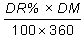This allows the quoted price to be expressed as a cash discount from the face value of the T-bill as follows:where

D = the cash discount;

DR% = discount rate percentage tendered;

F = the face amount of the bill;

DM = the number of days to maturity; and

360 = the convention for expressing the discount relative to a 360-day year.

Finally, the price of a T-bill is determined by subtracting the discount from the face value of the bill:Where P is price. This is what you would actually pay if you bought the T-bill.

You can convert the discount yield directly into the price using the formula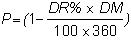Online, you can relate the Treasury bill information to the Treasury Calculator. You can access the interactive Treasury Calculator.The top, lefthand tab is Treasury Bills. The quoted price is entered into the field labeled Discount. Recall that quotes are provided in the form of an annualized discount rate percentage, DR%, called the discount yield. The quotation convention is relative to a face amount of \$100. The day count for the remaining life of the T-bill can be computed in the bottom part of the calculator and entered in the field labeled Days to Maturity.

By clicking the OK button, the T-bill calculator computes the price in dollars for a T-bill with a face amount equal to \$100. The bond equivalent yield for the T-bill is also automatically computed. In addition, the yield to maturity for the T-bill is computed assuming compounding on a daily basis.

Online you can access the interactive calculator immediately below:

Example for Interactive Calculator

Suppose the quoted discount is 4.12% for a 13-week (91-day) T-bill with a face value of \$1 million. What is the price, in terms of dollars, that you would have to pay for this T-bill?

Using the online calculator above enter this information as follows: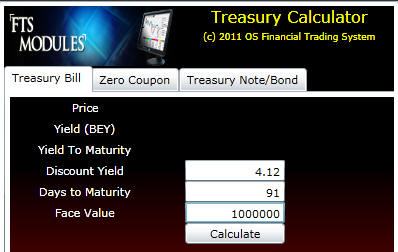Click on Calculate to see the answer.

The form of the bid is in terms of an implied cash discount from the face value, \$1 million, of the T-bill. This is computed in the following way: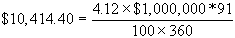Subtracting this discount from the face value gives the price:The T-bill market results reported in the financial press follow the convention of the Federal Reserve Bank of New York. The bank reports prices in its "Composite Quotations for U.S. Government Securities" every day. Prices in this list are for transactions exceeding \$1 million, obtained by polling five registered dealers in Treasuries. This list is subsequently reported the next day by the major financial newspapers and on the same day by market data services.

Primary auctions are quoted in the same way, which you will see by working through the next example.

Auction Report Example

Our goal is to understand how to translate bids in the Treasury auction, which are provided in terms of discounts from some face amount into actual contract values.

On Wednesday, April 20, 1994, The Wall Street Journal reported the (average) results from the Monday Treasury-bill auction. Face value amounts from \$10,000 to \$1 million were sold at an average discount from face value at 3.76% for 13 weeks and 4.21% for 26 weeks.

Note how much interest rates have changed today!

The average cash discount for the 13-week issue was:The average cash discount for the 26-week issue was:As a result, the average price paid for a 13-week issue with a \$1 million face value was:Similarly, the average price paid for a 26-week issue with a \$1 million face value was: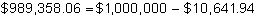Interactive Online Exercise:  Compare to Today

WSJ reported March 7, 2011 that the March 17, 2011 Treasury Bill quotes was bid = 0.075 and the ask = 0.070.  The asked yield was reported to be 0.071.  Treasury Bill rates were very low in 2011 and the you can verify interactively that by entering this information into the online calculator for \$1,000,000 provides the appropriate numbers.

You can compare those yields to the yields today by clicking on "Recent CMT" which downloads the current yields on a Treasury with exactly 3 months to maturity.

Reported T-bill Yields

Reported Treasury yields are a very closely watched statistic. The reason is that T-bill yields are used to construct the current yield curve, which in turn affects the price of many other securities with cash flows that extend over time.

To compare yields across different times to maturity, you need to know the convention used for quoting the yield in a particular market. For T-bills, the yield is reported on a coupon equivalent basis or, as it is sometimes referred to, the bond-equivalent yield, computed from the ask price.

This yield is computed as simple or fractional interest, as opposed to compound interest. The convention for computing the bond-equivalent yield depends upon whether the remaining life of the T-bill is greater than 6 months or less than 6 months. The best way to understand the conventions is to work through the following examples.

Bond Equivalent Yields for 3- and 6-Month T-Bills or 12 Month T-Bills with 6 Months or Less Remaining Life

Assume that the ask price in the secondary market is equal to the price in the cash value of the bid example completed earlier. That is, let us assume that the ask equals the bid of \$989,585.60, and the face value is \$1 million.

The market convention for quoting the bond equivalent yield for 3- and 6-month T-bills is to express the bond equivalent yield relative to the price and a 365-day year as follows: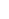where F is the face amount and DM is the number of days to maturity. The reported yield for our example on a coupon equivalent basis is thus:This simple interest-based yield in general closely approximates, but does not exactly equal, the yield to maturity (i.e., the compounded rate) for the T-bill. You can verify that the annualized spot interest rate or yield to maturity (compounded daily) that equates the present value of the face amount to the observed ask price is 4.20%. Over a short time horizon, the two differences are the same.

When the remaining life of a 12-month T-bill is less than 6 months, then the bond equivalent yield is reported in the same way, as a 3- or 6-month T-bill. For example, at the close of markets for Monday, February 13, 1995, the following data was reported for the 12-month T-bill maturing on June 29, 1995:

 Contract Bid Ask Yield June 29, 1995 5.88 5.86 6.07

(Source: The Wall Street Journal, Tuesday, February 14, 1995)

Bond Tutor’s calculator automatically computes the BEY using the method that matches the remaining life of the T-bill.

By using the Online Treasury calculator, you can verify that in the current example, because the remaining life of this 12-month T-bill is less than 6 months, the BEY is computed in the same way as for any 3- or 6-month T-bill.  Below assume that the Days to Maturity equals 91.

That is, the calculated and reported values agree (e.g., BEY = 4.2212%).

Bond Equivalent Yields for 12-Month T-Bills with a Remaining Life Greater than 6 Months

At the close of markets for Friday, September 16, 1994, the following data was reported for the 12-month T-bills maturing on June 29, 1995 is:

 Contract Bid Ask Yield June 29, 1995 5.29 5.25 5.50

(Source: Investor’s Business Daily, Monday, September 19, 1994)

The reported number under the yield column is the Bond Equivalent Yield for the 12-month T-bill. The market convention for the 12-month T-bill that has a remaining life exceeding 6 months is different to the convention for the 3- and 6- month T-bills. It is as follows: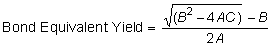where: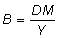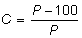DM = Days to maturity

P = Ask price relative to \$100 face value

Y = Total number of days in the year following the issue date (in Bond Tutor, the calculator assumes a 365-day calender year)

For the June 29th contract above, these values are:

 A 0.140411 B 0.780822 C -0.04336 DM 285 BEY 0.055

Using the calculator in Bond Tutor, you can verify that the computed BEY matches this figure.

For further discussion of interest rates, see Chapter 1, Topic 1.4., The Calculation of Interest.